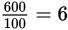# SAT Math Multiple Choice Question 839: Answer and Explanation

### Test Information

Question: 839

14. An oil tank has two pipes connected to it. If the tank is empty, Pipe A can fill itin 2 hours. If the tank is full, Pipe B can empty it in 3 hours. If both pipes areactivated at the same time when the tank is empty, how many hours will it take forthe tank to be filled to 60% of its capacity?

• A. 2.4
• B. 3.6
• C. 6
• D. 60

Getting to the Answer: Pick a number for the capacity of the oil tank, choosing one that divides nicely by 2 and 3. Let the capacity of the tank equal 600 gallons. Given that Pipe A can fill the tank in 2 hours, its rate is 600 ÷ 2 = 300 gallons per hour. Likewise, Pipe B can empty the tank in 3 hours, so its rate is 600 ÷ 3 = 200 gallons per hour. If Pipe A adds 300 gallons per hour while Pipe B removes 200 gallons per hour, then a net of 100 gallons of oil will be added to the tank each hour. At this rate, it will takehours to fill the tank. But wait-this isn't the answer. The question asks how long it will take to fill 60% of the tank, not the whole tank. Multiply the time by 0.6 to get the final answer: (6)(0.6) = 3.6 hours, choice (B).